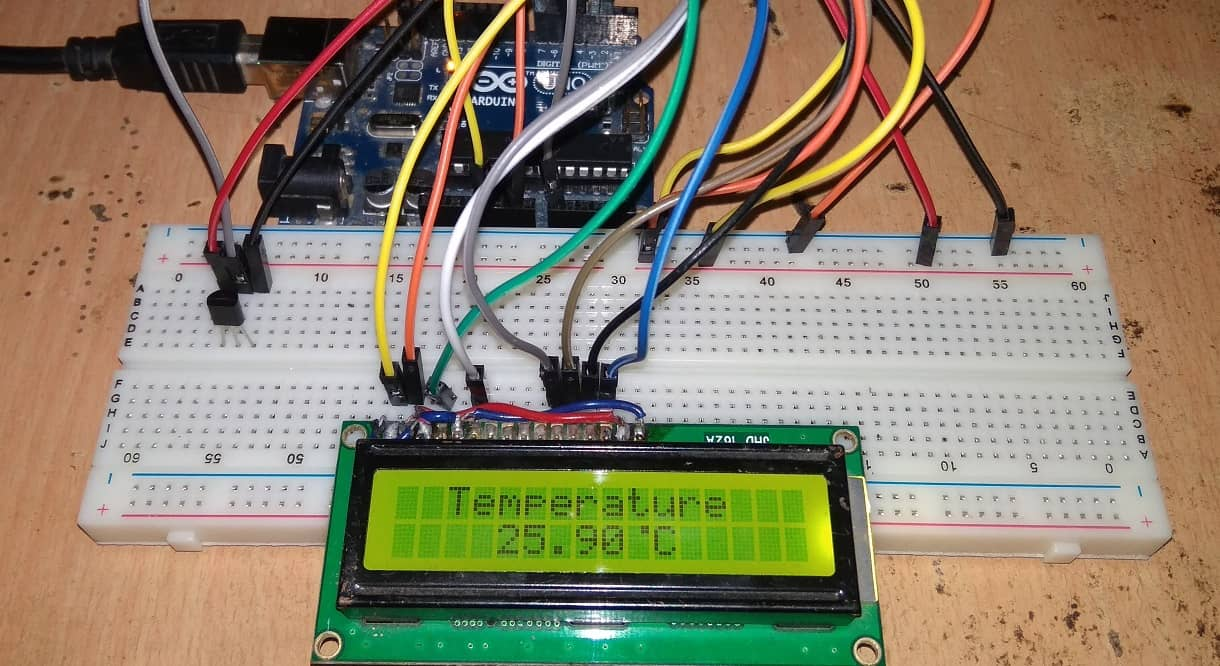Digital Thermometer Using Arduino & LM35 Temperature Sensor(Last Updated On: February 25, 2019)

Digital Thermometer Using Arduino & LM35 Temperature Sensor:

In this project we interfaced LM35 Temperature Sensor with Arduino to design digital thermometer. The measured temperature will be directly displayed on 16*2 LCD. LM35DZ is capable of reading the temperature in Centigrade scale. The output voltage of the sensor is directly proportional to the temperature in centigrade. LM35 can be used in the range of -55°C to +150°C with +/- 0.75°C accuracy. So lets learn how to design Digital Thermometer Using Arduino & LM35 Temperature Sensor.

Components Required:

We need following components for designing digital thermometer.

```1. Arduino UNO Board
2. LM35DZ Temperature Sensor
3. 16*2 LCD Display
5. Connecting Wires```

LM35 Temperature Sensor:

Introduction:

The LM35 series are precision integrated-circuit temperature devices with an output voltage linearly-proportional to the Centigrade temperature. The LM35 device has an advantage over linear temperature sensors calibrated in Kelvin, as the user is not required to subtract a large constant voltage from the output to obtain convenient Centigrade scaling. The LM35 device does not require any external calibration or trimming to provide typical accuracies of ±¼°C at room temperature and ±¾°C over a full −55°C to 150°C temperature range.Lower cost is assured by trimming and calibration at the wafer level. The low-output impedance, linear output, and precise inherent calibration of the LM35 device makes interfacing to readout or control circuitry especially easy. The device is used with single power supplies, or with plus and minus supplies. As the LM35 device draws only 60 µA from the supply, it has very low self-heating of less than 0.1°C in still air.

Features:

1. Calibrated directly in Degree Celsius (Centigrade)
2. Linear at 10.0 mV/°C scale factor
3. 0.5°C accuracy guarantee-able (at a25°C)
4. Rated for full -55°C to a 150°C range
5. Suitable for remote applications
6. Low cost due to wafer-level trimming
7. Operates from 4 to 30 volts
8. Less than 60 mA current drain
9. Low self-heating, 0.08°C instill air
10. Non-linearity only 0.25°C typical
11. Low impedance output, 0.1Ωfor 1 mA load

Working:

In order to understand the working principle of lm35 temperature sensor we have to understand the linear scale factor. In the features of lm35 it is given to be +10 mills volt per degree centigrade. It means that with increase in output of 10 mills volt by the sensor vout pin the temperature value increases by one. For example if the sensor is outputting 100 mills volt at vout pin the temperature in centigrade will be 10 degree centigrade. The same goes for the negative temperature reading. If the sensor is outputting -100 mills volt the temperature will be -10 degree Celsius.The circuit diagram is shown above. Briefly, there are two transistors in the center of the drawing. One has ten times the emitter area of the other. This means it has one tenth of the current density, since the same current is going through both transistors. This causes a voltage across the resistor R1 that is proportional to the absolute temperature, and is almost linear across the range we care about. The “almost” part is taken care of by a special circuit that straightens out the slightly curved graph of voltage versus temperature. The amplifier at the top ensures that the voltage at the base of the left transistor (Q1) is proportional to absolute temperature (PTAT) by comparing the output of the two transistors.

The amplifier at the right converts absolute temperature (measured in Kelvin) into either Fahrenheit or Celsius, depending on the part (LM34 or LM35). The little circle with the “i” in it is a constant current source circuit. The two resistors are calibrated in the factory to produce a highly accurate temperature sensor. The integrated circuit has many transistors in it — two in the middle, some in each amplifier, some in the constant current source, and some in the curvature compensation circuit. All of that is fit into the tiny package with three leads

Circuit Diagram & Connections:

First of all, we will make the connection to LM35 Temperature Sensor. Connect the 1st pin of LM35 to 5V of Arduino UNO and 3rd Pin to GND. Similarly connect the 2nd pin to Analog input pin A0 of Arduino UNO

Now we will connect the 16×2 LCD to the Arduino.
1. Connect pin 1,3,5,16 of LCD to the GND.
2. Connect pin 2,15 of LCD to the VCC (5V).
3. Connect pin 4 of LCD to pin D8 of Arduino.
3. Connect pin 6 of LCD to pin D9 of Arduino.
3. Connect pin 11 of LCD to pin D10 of Arduino.
3. Connect pin 12 of LCD to pin D11 of Arduino.
3. Connect pin 13 of LCD to pin D12 of Arduino.
3. Connect pin 14 of LCD to pin D13 of Arduino.

Source Code/Program:

The source code for Digital Thermometer Using Arduino & LM35 Temperature Sensor is given below. Copy this code and paste it in your Arduino IDE and the upload it to the Arduino Board.

```#include<LiquidCrystal.h>
LiquidCrystal lcd(8,9,10,11,12,13);
#define sensor A0
byte degree =
{
0b00011,
0b00011,
0b00000,
0b00000,
0b00000,
0b00000,
0b00000,
0b00000
};
void setup()
{
lcd.begin(16,2);
lcd.createChar(1, degree);
lcd.setCursor(0,0);
lcd.print(" Digital ");
lcd.setCursor(0,1);
lcd.print(" Thermometer ");
delay(2000);
lcd.clear();
}
void loop()
{
/*---------Temperature-------*/
delay(10);

/*------Display Result------*/
lcd.clear();
lcd.setCursor(2,0);
lcd.print("Temperature");
lcd.setCursor(4,1);
lcd.print(temperature);
lcd.write(1);
lcd.print("C");
delay(1000);
}```

Video Demonstration & Explanation:

Watch this video tutorial that explains all about Digital Thermometer Using Arduino & LM35 Temperature Sensor.

Recommended For You

1.jesima says:
1.Alex Newton says: